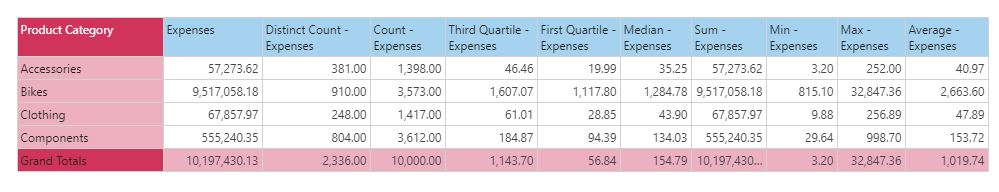#Maximum (PQL)

Executes maximum aggregation on a column of data from the model. The column needs to have numerical data for it to be functional.

• Returned Output: Numeric
• Library: PQL \ Granular \ Aggregate
• Version: 2018.00.000
• Compatibility: Pyramid Query Language (PQL) data sources

#### Syntax

Maximum( <Measure> )

###### Syntax Alternatives

Max( <Measure> )

* Click on the function's arguments above for more details on the input values.

• The "measure" column needs to be the name of the column in the model [table].[column name] or it can be an existing measure in the model [measures].[column name].
• For details on how to employ and use this function see the granular calculation overview.
##### Different Function types
• This function is like the SQL max function; calculating the maximum of a column of data in your query or model.
• If you wish to find the maximum for the elements in a specified SET or LIST from a hierarchy, use this semantic function.
• To use a simple maximum mathematical operation on a list of numbers, use the Common library function.

#### Examples

To create a new measure that is the sum of expenses in the model, using the sample demo model:

Maximum( [data].[Expenses] )

or:

Max( [measures].[data Expenses] )

The mechanics of this function are shown below. This grid says that the maximum expense across all 'Accessory' transactions is \$252.00. This was calculated by looking at every row for accessory transactions and finding the one with the highest value. This produces a different result to the Max([LIST]) function which would find the maximum value from the list of items presented instead.